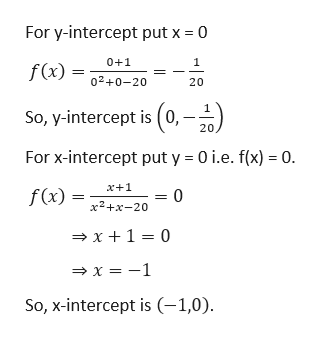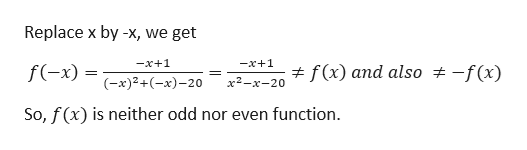# Can you please help with the following problem: f(x)=x+1/x^2+x-20 a. Find the x and y interceptsb. Is the function even, odd, or neither?c. Find the vertical asymptotesd. Find the horizontal or slant asymptotes if any.e. Graph the asymptotes, if any, and use all the information to connect the points and graph f(x)d. What is the domain and range?Thank you!

Question
6 views

f(x)=x+1/x^2+x-20

a. Find the x and y intercepts

b. Is the function even, odd, or neither?

c. Find the vertical asymptotes

d. Find the horizontal or slant asymptotes if any.

e. Graph the asymptotes, if any, and use all the information to connect the points and graph f(x)

d. What is the domain and range?

Thank you!

check_circle

Step 1

As per our policy I am solving only 1st three subparts and for the subparts please post the question again separately.

Given,

Step 2

Part ahelp_outlineImage TranscriptioncloseFor y-intercept put x 0 0+1 f(x) 02+0-20 20 So, y-intercept is (0,-- 20, For x-intercept put y 0 i.e. f(x) 0. x+1 f(x) x2+x-20 x1 0 x-1 So, x-intercept is (-1,0) fullscreen
Step 3

Part b

...help_outlineImage TranscriptioncloseReplace x by -x, we get -x+1 -x+1 f(x) and also f(-x) -f(x) (x)2+(x)-20 х2—х-20 So, f(x) is neither odd nor even function fullscreen

### Want to see the full answer?

See Solution

#### Want to see this answer and more?

Solutions are written by subject experts who are available 24/7. Questions are typically answered within 1 hour.*

See Solution
*Response times may vary by subject and question.
Tagged in

### Other